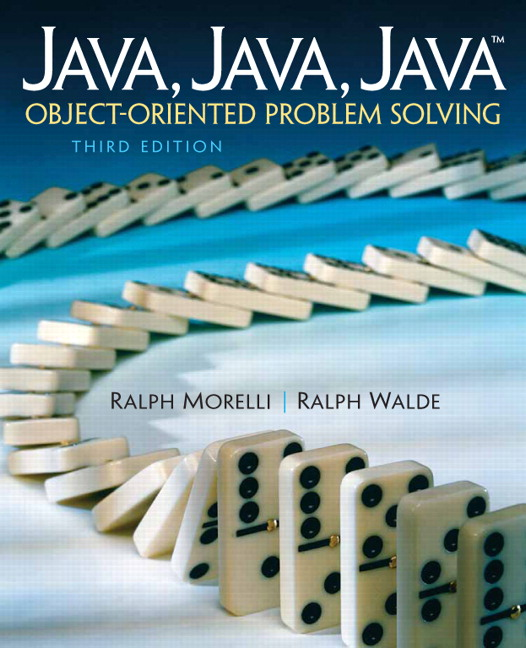# Java, Java, Java: Object-Oriented Problem Solving, 2022E

## Section5.6From the Java Library: java.lang.Math

The java.lang.Math class provides many common mathematical functions that will prove useful in performing numerical computations. As an element of the java.lang package, it is included implicitly in all Java programs. Table 5.6.1 lists some of the most commonly used Math class methods.

### Subsection5.6.1Static Class Elements

The Math is somewhat exceptional in terms of what we’ve already learned about Java classes. Here is an outline of how it is defined:
public final class Math    // Final, can't subclass
{   private Math() {}      // Private, can't invoke
...
public static native double sqrt(double a)
throws ArithmeticException;
}

Notice that the class is declared public final. This means that it can be accessed (public) but it cannot be extended or subclassed (final). Notice that its default constructor is declared private. This prevents this class from being instantiated — i.e., there can be no Math objects.
The idea of a class that cannot be subclassed and cannot be instantiated may seem a little strange at first. The justification for it here is that it provides a convenient and efficient way to introduce helpful math functions into the Java language.
Notice also that the sqrt() method is declared static, as are all Math class methods:
public static native double sqrt(double a)
throws ArithmeticException;

This means that when we want to use a Math method, we have to call it using the class name. For example, we would calculate $$2^4$$ as Math.pow(2,4), which evaluates to 16. Similarly, we compute the square root of 225.0 as Math.sqrt(225.0), which evaluates to 15.0.
Defining the Math class in this way makes it easy to use its methods, because you don’t have to create Math objects. It is also a very efficient design because java.lang.Math methods are loaded into memory at the beginning of your program’s execution, and they persist in memory throughout your program’s lifetime.
Random numbers are very useful in coding, for example in games. Math.random() returns a random number between 0.0-0.99. We often need to move the random number to be in a certain range, for example to get a random number between 1 to 10. (int)(Math.random()* range) + minmoves the random number into a range starting from a minimum number. The range is the (max number - min number + 1).
// returns a random number between 0-.99
double randDec = Math.random();
// returns a random number between 1 and 10
int randNum = (int)(Math.random()* 10) + 1;


#### Activity5.6.1.

Run the code below to see the Math methods being used. Run it several times to see how the random value changes each time. How could you change the code to return a random integer from 5 to 15?
In general, static class elements — methods and variables — are useful when we need elements that don’t depend on the existence of objects of a class. For example, consider the following class definition:
public Widget
{
public static nWidgets = 0;
public Widget()
{
nWidgets++;
}
}

Here we have a public static variable, nWidgets that is meant to keep a count of the number of widgets created. The count is incremented every time the constructor is called — i.e., every time a widget is created. Obviously, before the first widget is created we want to be able to say that the count is 0. We can do so by accessing Widget.nWidgets.
We will see other occasions to use the static and final qualifiers in subsequent chapters.